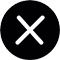News
NEWS
The size and capacity of dry containers commonly used internationally

1. The external dimensions are 20 feet X 8 feet X 8 feet 6 inches, which is referred to as a 20-foot container;

2. 40 feet X 8 feet X 8 feet 6 inches, referred to as 40 feet container;

3. 40 feet X 8 feet X 9 feet 6 inches, referred to as 40 feet tall cabinet (used more in recent years).

20-foot container: The internal volume is 5.94 meters X 2.34 meters X 2.39 meters, the gross weight of the goods is up to 28.25 tons, and the volume is 33 cubic meters.

40-foot container: The internal volume is 12.06 meters X 2.34 meters X 2.39 meters, the gross weight of the distribution is up to 28.8 tons, and the volume is 67 cubic meters.

40-foot high cabinet: The internal volume is 12.06 meters X 2.34 meters X 2.69 meters. The gross weight of the goods is up to 30.2 tons, and the volume is 76 cubic meters.

45-foot high cabinet: The internal volume is: 13.5 meters X 2.34 meters X 2.71 meters, the gross weight of the distribution is up to 27.7 tons, and the volume is 85 cubic meters.

20-foot open top cabinet: The internal volume is 5.89 meters X 2.32 meters X 2.31 meters, the gross weight is 20 tons, and the volume is 31.5 cubic meters.

40-foot open top cabinet: The internal volume is 12.01 meters X 2.33 meters X 2.15 meters, the gross weight of the distribution is 30.4 tons, and the volume is 65 cubic meters.

20-foot flat-bottom container: internal volume 5.85 meters X 2.23 meters X 2.15 meters, gross weight 23 tons, volume 28 cubic meters.

40-foot flat-bottom container: internal volume 12.05 meters X 2.12 meters X 1.96 meters, distribution gross weight 36 tons, volume 50 cubic meters.

FCL Booking from Shanghai  to U.S.